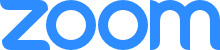#Modelling and Visualising Pandemic Data - Shared screen with speaker viewAmlesh Kanekar
10:06
https://rviews.rstudio.com/2020/03/05/covid-19-epidemiology-with-r/Aishwarya Sarangi
13:17
I am unable to hear the discussion.Meghnad Desai Academy of Economics
16:48
join again?Aishwarya Sarangi
19:15
Thanks. :)Meghnad Desai Academy of Economics
22:41
estimr codeRupeesha Galhotra
26:11
Error: unexpected symbol in:"out <- ode(y = init, times = Day, func = SIR, parms = parameters)fit <- out[ , 3]sum"Payal Ghose
29:20
Same errorMeghnad Desai Academy of Economics
29:57
install.packages("deSolve")Meghnad Desai Academy of Economics
30:05
library(deSolve)Rupeesha Galhotra
31:18
out <- ode(y = init, times = Day, func = SIR, parms = parameters)Rupeesha Galhotra
31:50
init <- c(S = N-Infected, I = Infected,R = 0)RSS <- function(parameters) {names(parameters) <- c("beta", "gamma")out <- ode(y = init, times = Day, func = SIR, parms = parameters)fit <- out[ , 3]sum((Infected - fit)^2)}Rupeesha Galhotra
32:59
with(par, {dS <- -beta/N * I * SdI <- beta/N * I * S- gamma * IdR <- gamma * Ilist(c(dS,dI, dR))})}install.packages("deSolve")library(deSolve)init <- c(S = N-Infected, I = Infected,R = 0)RSS <- function(parameters) {names(parameters) <- c("beta", "gamma")out <- ode(y = init, times = Day, func = SIR, parms = parameters)fit <- out[ , 3]sum((Infected - fit)^2)}Opt <- optim(c(0.5,0.5), RSS, method ="L-BFGS-B" ,lower = c(0.01, 0.01), upper = c(1, 1)) # optimize with some sensible conditionsOpt\$message##  "CONVERGENCE: REL_REDUCTION_OF_F <= FACTR*EPSMCH"Payal Ghose
34:59
yesRupeesha Galhotra
35:31
solvedMeghnad Desai Academy of Economics
35:37
Mr. Dhume, replace your code with this:Meghnad Desai Academy of Economics
35:48
init <- c(S = N-Infected, I = Infected,R = 0)RSS <- function(parameters) { names(parameters) <- c("beta", "gamma")out <- ode(y = init, times = Day, func = SIR, parms = parameters)fit <- out[ , 3]sum((Infected - fit)^2)}Opt <- optim(c(0.5,0.5), RSS, method ="L-BFGS-B" ,lower = c(0.01, 0.01), upper = c(1, 1)) # optimize with some sensible conditionsOpt\$messageMeghnad Desai Academy of Economics
36:20
sum((Infected - fit)^2)} this has to go on the next lineMeghnad Desai Academy of Economics
36:28
sure.Shubhangi Sharma
36:49
Sir, what is the method underlying this optimation?Meghnad Desai Academy of Economics
36:52
there is only one line that is wrong. do correct if possible so you can do it with usPooja Patki
01:02:43
are you sure you have the latest R version? I had to redownload to run some codesMekhala Singhal
01:30:00
Sir but there has been proof of reinfection, right? In that case where would this omdel apply?Mekhala Singhal
01:30:06
modelMekhala Singhal
01:31:21
Thank you!Amlesh Kanekar
01:40:00
Thank you very much Dr. Hatekar and Fatima!!!!Meghnad Desai Academy of Economics
01:42:18
sure DivyaMeghnad Desai Academy of Economics
01:42:28